# How to Find Standard Deviation in R?

Published on August 3, 2022By Prajwal CN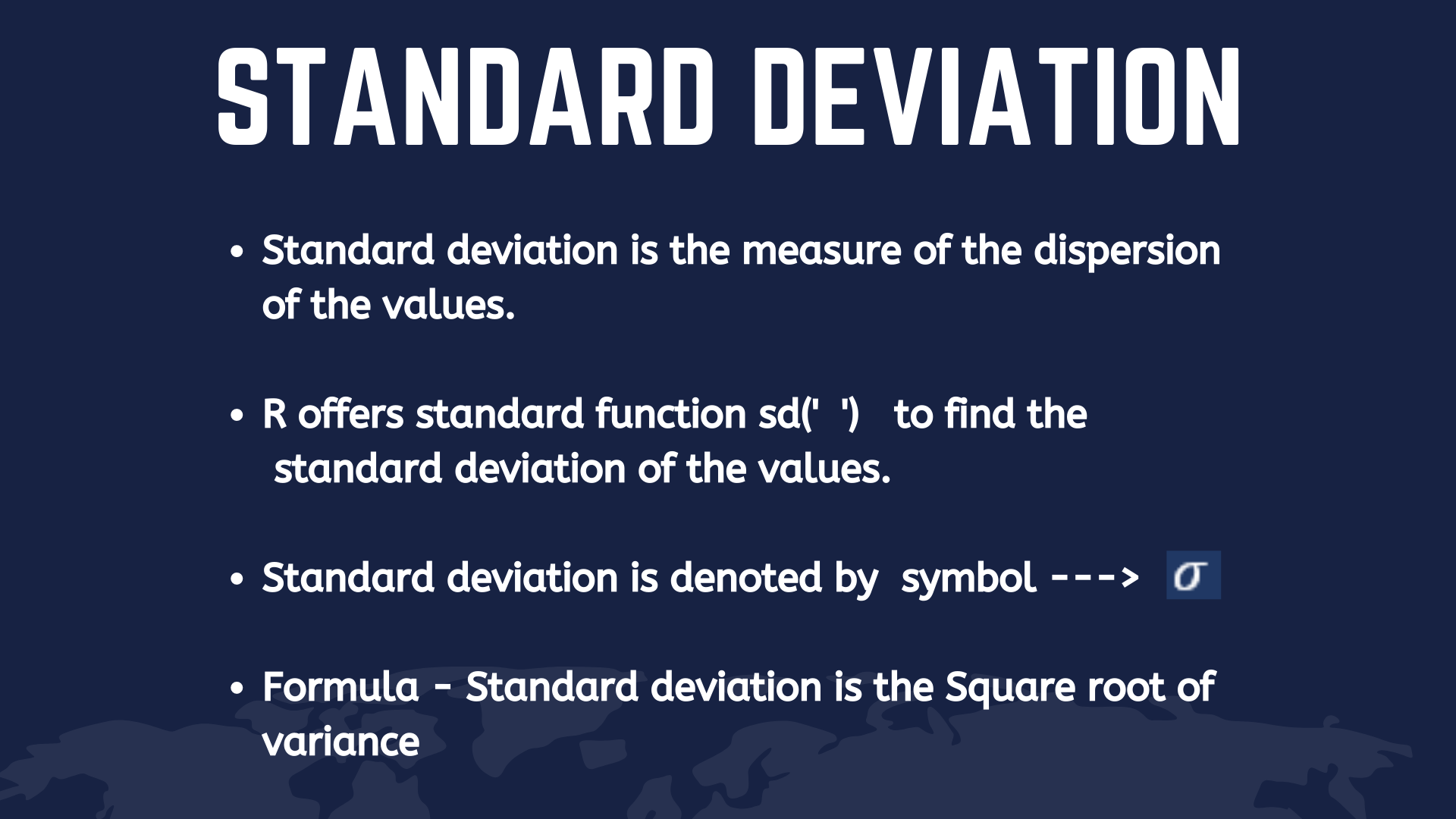While we believe that this content benefits our community, we have not yet thoroughly reviewed it. If you have any suggestions for improvements, please let us know by clicking the “report an issue“ button at the bottom of the tutorial.

Being a statistical language, R offers standard function sd(’ ') to find the standard deviation of the values.

## So what is the standard deviation?

• ‘Standard deviation is the measure of the dispersion of the values’.
• The higher the standard deviation, the wider the spread of values.
• The lower the standard deviation, the narrower the spread of values.
• In simple words the formula is defined as - Standard deviation is the square root of the ‘variance’.

## Importance on Standard deviation

Standard deviation is very popular in the statistics, but why? the reasons for its popularity and its importance are listed below.

• Standard deviation converts the negative number to a positive number by squaring it.
• It shows the larger deviations so that you can particularly look over them.
• It shows the central tendency, which is a very useful function in the analysis.
• It has a major role to play in finance, business, analysis, and measurements.

Before we roll into the topic, keep this definition in your mind!

Variance - It is defined as the squared differences between the observed value and expected value.

## Find the Standard deviation in R for values in a list

In this method, we will create a list ‘x’ and add some value to it. Then we can find the standard deviation of those values in the list.

`````` x <- c(34,56,87,65,34,56,89)    #creates list 'x' with some values in it.

sd(x)  #calculates the standard deviation of the values in the list 'x'
``````

Output —> 22.28175

Now we can try to extract specific values from the list ‘y’ to find the standard deviation.

`````` y <- c(34,65,78,96,56,78,54,57,89)  #creates a list 'y' having some values

data1 <- y[1:5] #extract specific values using its Index

sd(data1) #calculates the standard deviation for Indexed or extracted values from the list.
``````

Output —> 23.28519

## Finding the Standard deviation of the values stored in a CSV file

In this method, we are importing a CSV file to find the standard deviation in R for the values which are stored in that file.

``````readfile <- read.csv('testdata1.csv')  #reading a csv file

sd(data2)                              #calculates the standard deviation
``````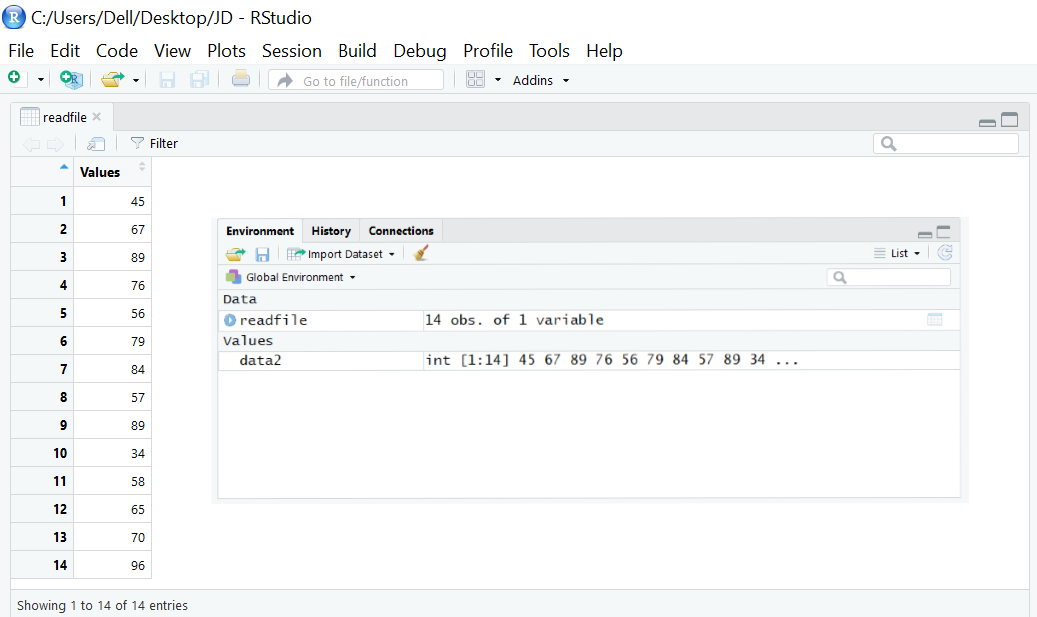Output —> 17.88624

## High and Low Standard Deviation

In general, The values will be so close to the average value in low standard deviation and the values will be far spread from the average value in the high standard deviation.

We can illustrate this with an example.

``````x <- c(79,82,84,96,98)
mean(x)
--->  82.22222
sd(x)
--->  10.58038
``````

To plot these values in a bar graph using in R, run the below code.

To install the ggplot2 package, run this code in R studio.

-–> install.packages(“ggplot2”)

``````library(ggplot2)

values <- data.frame(marks=c(79,82,84,96,98), students=c(0,1,2,3,4,))
marks students
1    79        0
2    82        1
3    84        2
4    96        3
5    98        4
x <- ggplot(values, aes(x=marks, y=students))+geom_bar(stat='identity')
x                             #displays the plot
``````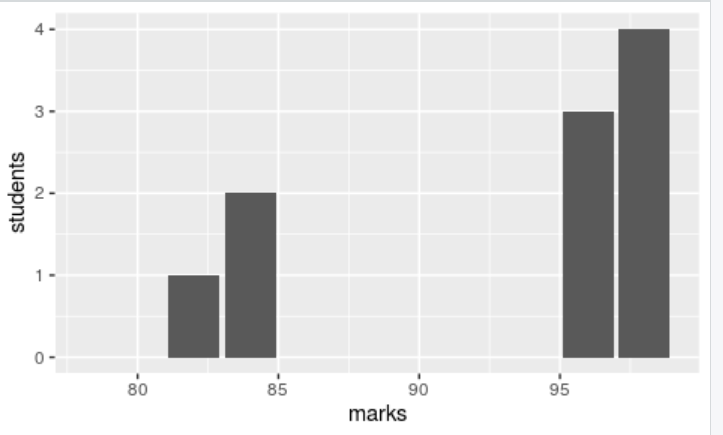In the above results, you can observe that most of the data is clustering around the mean value(79,82,84) which shows that it is a low standard deviation.

Illustration for high standard deviation.

``````y <- c(23,27,30,35,55,76,79,82,84,94,96)
mean(y)
---> 61.90909
sd(y)
---> 28.45507
``````

To plot these values using a bar graph in ggplot in R, run the below code.

``````library(ggplot2)

values <- data.frame(marks=c(23,27,30,35,55,76,79,82,84,94,96), students=c(0,1,2,3,4,5,6,7,8,9,10))
marks students
1    23        0
2    27        1
3    30        2
4    35        3
5    55        4
6    76        5
x <- ggplot(values, aes(x=marks, y=students))+geom_bar(stat='identity')
x                             #displays the plot
``````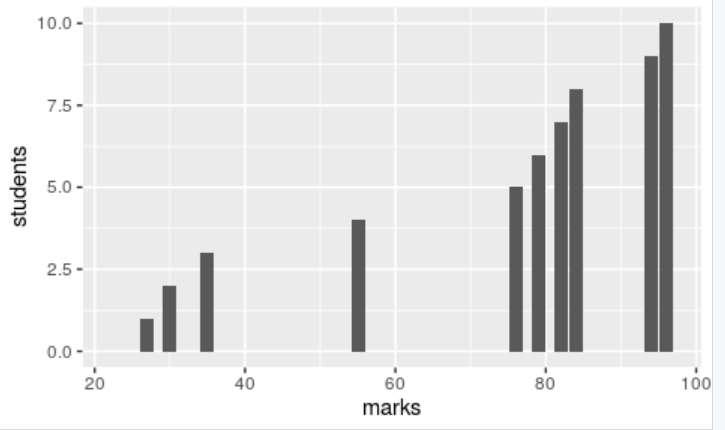In the above results, you can see the widespread data. You can see the least score of 23 which is very far from the average score 61. This is called the high standard deviation

By now, you got a fair understanding of using the sd(’ ') function to calculate the standard deviation in the R language. Let’s sum up this tutorial by solving simple problems.

## Example #1: Standard Deviation for a List of Even Numbers

Find the standard deviation of the even numbers between 1-20 (exclude 1 and 20).

Solution: The even numbers between 1 to 20 are,

-–> 2, 4, 6, 8, 10, 12, 14, 16, 18

Lets find the standard deviation of these values.

``````x <- c(2,4,6,8,10,12,14,16,18)  #list of even numbers from 1 to 20

sd(x)                           #calculates the standard deviation of these
values in the list of even numbers from 1 to 20
``````

Output —> 5.477226

## Example #2: Standard Deviation for US Population Data

Find the standard deviation of the state-wise population in the USA.

For this, import the CSV file and read the values to find the standard deviation and plot the result in a histogram in R.

``````df<-read.csv("population.csv")      #reads csv file
data<-df\$X2018.Population           #extarcts the data from population
column
mean(data)                          #calculates the mean

View(df)                            #displays the data
sd(data)                            #calculates the standard deviation
``````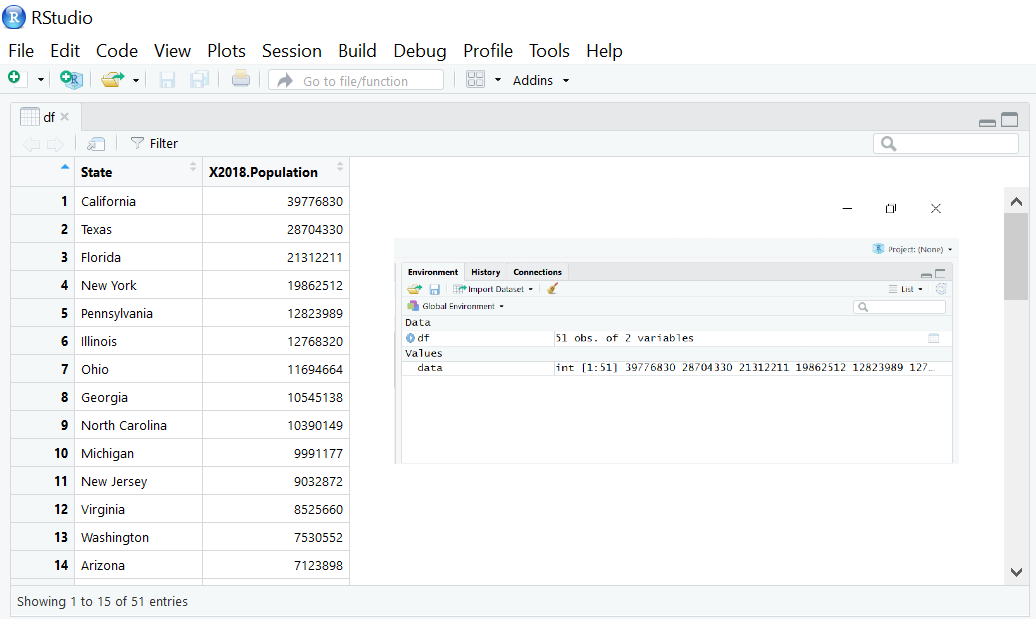Output ----> mean = 6432008, Sd = 7376752

## Conclusion

Finding the standard deviation of the values in R is easy. R offers standard function sd(’ ') to find the standard deviation. You can create a list of values or import a CSV file to find the standard deviation.

Important: Don’t forget to calculate the standard deviation by extracting some values from a file or a list through indexing as shown above.

Use the comment box to post any kind of doubts regarding the sd(’ ') function in R. Happy learning!!!

Thanks for learning with the DigitalOcean Community. Check out our offerings for compute, storage, networking, and managed databases.Prajwal CN

author

#### Still looking for an answer?

Ask a questionSearch for more help

JournalDev
DigitalOcean EmployeeApril 12, 2021

hey! this was help full but how to find sd for a grouped frequency data distribution for eg a table like this x f 5-10 12 10-20 28 20-30 65 30-40 121 40-50 175 50-60 198 60-70 176 70-80 120 80-90 66 90-100 27 100-115 9 115-120 3 … what to do after making them into a data.frame()

- Saptharishee M

Click below to sign up and get \$200 of credit to try our products over 60 days!

### Popular Topics##### Hollie's Hub for Good

Working on improving health and education, reducing inequality, and spurring economic growth? We’d like to help.##### Become a contributor

You get paid; we donate to tech nonprofits.

##### Welcome to the developer cloud

DigitalOcean makes it simple to launch in the cloud and scale up as you grow – whether you’re running one virtual machine or ten thousand.© 2023 DigitalOcean, LLC.